# Selina Solutions Concise Maths Class 10 Chapter 21 Trigonometrical Identities Exercise 21(B)

More problems on proving trigonometrical identities are contained in this exercise. It is recommended that students build a strong grip over the concepts as it forms the foundation of higher class Mathematics. The Selina Solutions for Class 10 Maths is the apt resource for students to clarify doubts and develop confidence in solving problems. The solutions to the Concise Selina Solutions for Class 10 Maths Chapter 21 Trigonometrical Identities Exercise 21(B) are accessible in PDF format, provided in the links given below.

## Selina Solutions Concise Maths Class 10 Chapter 21 Trigonometrical Identities Exercise 21(B) Download PDF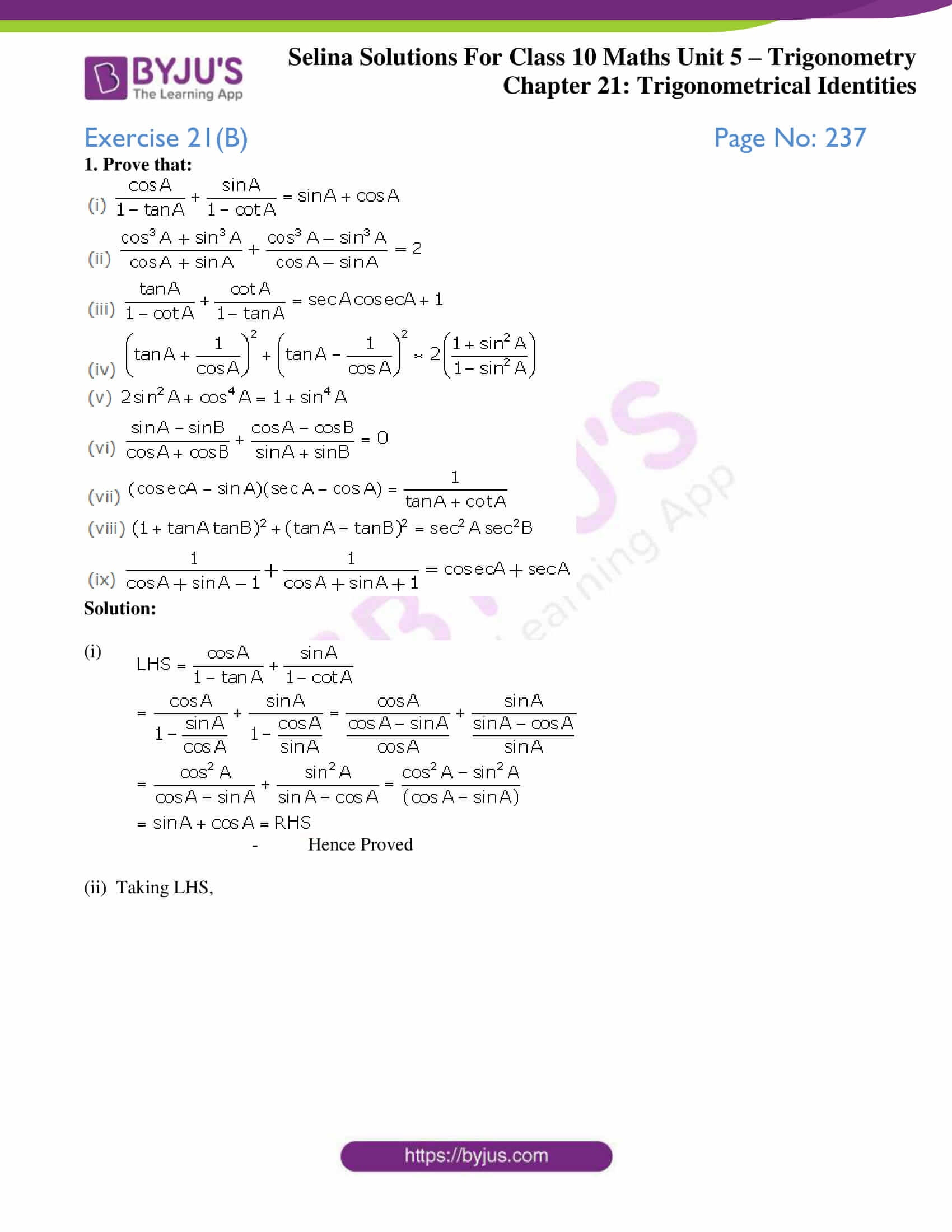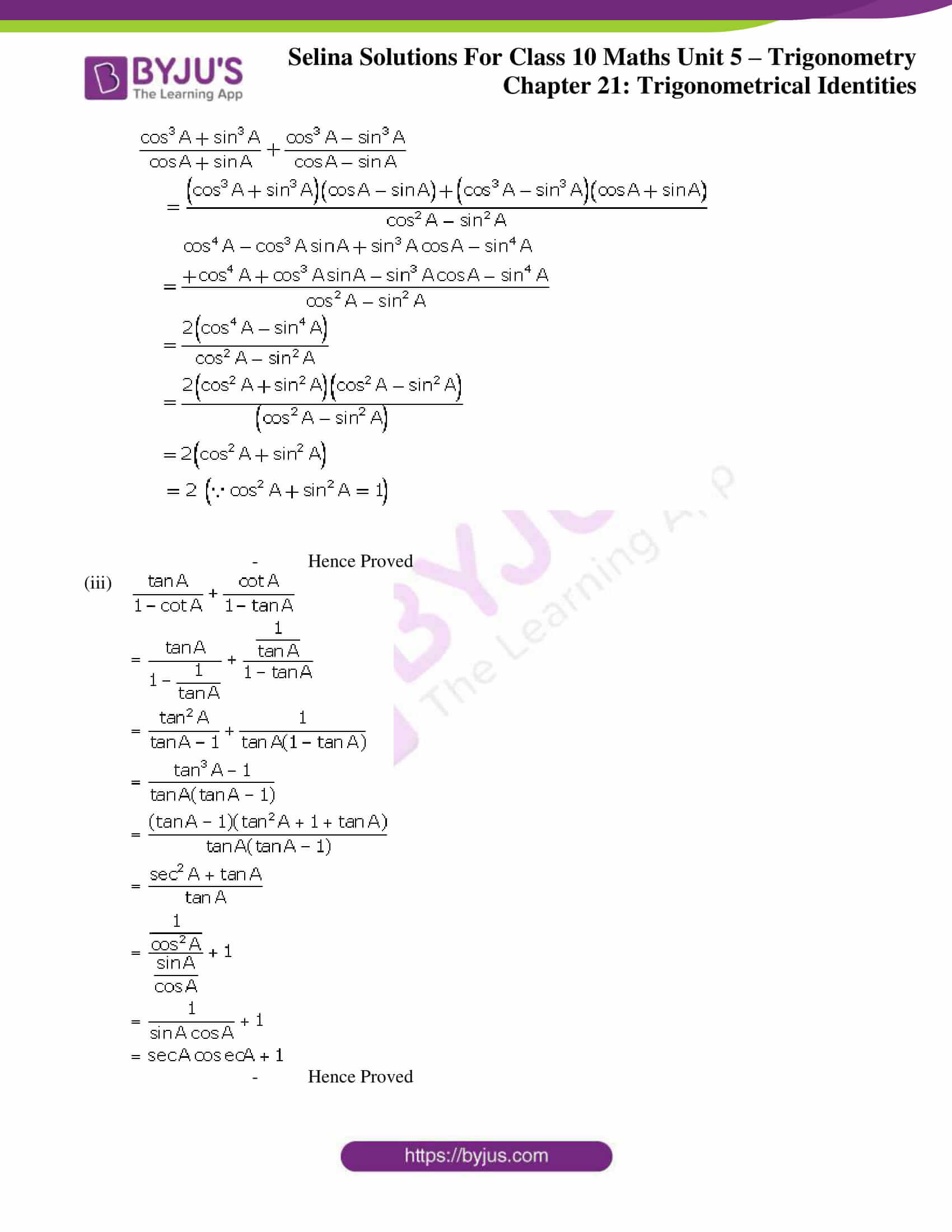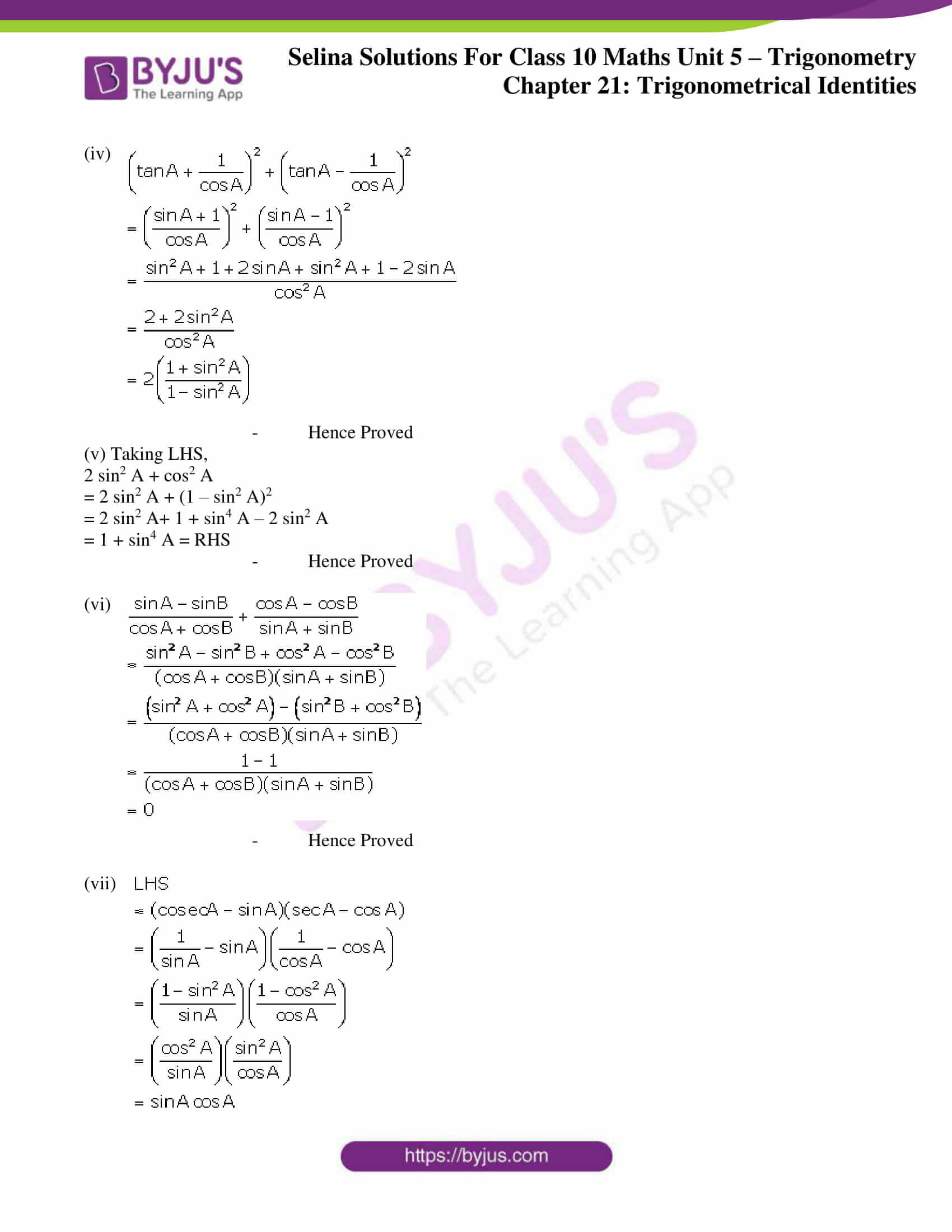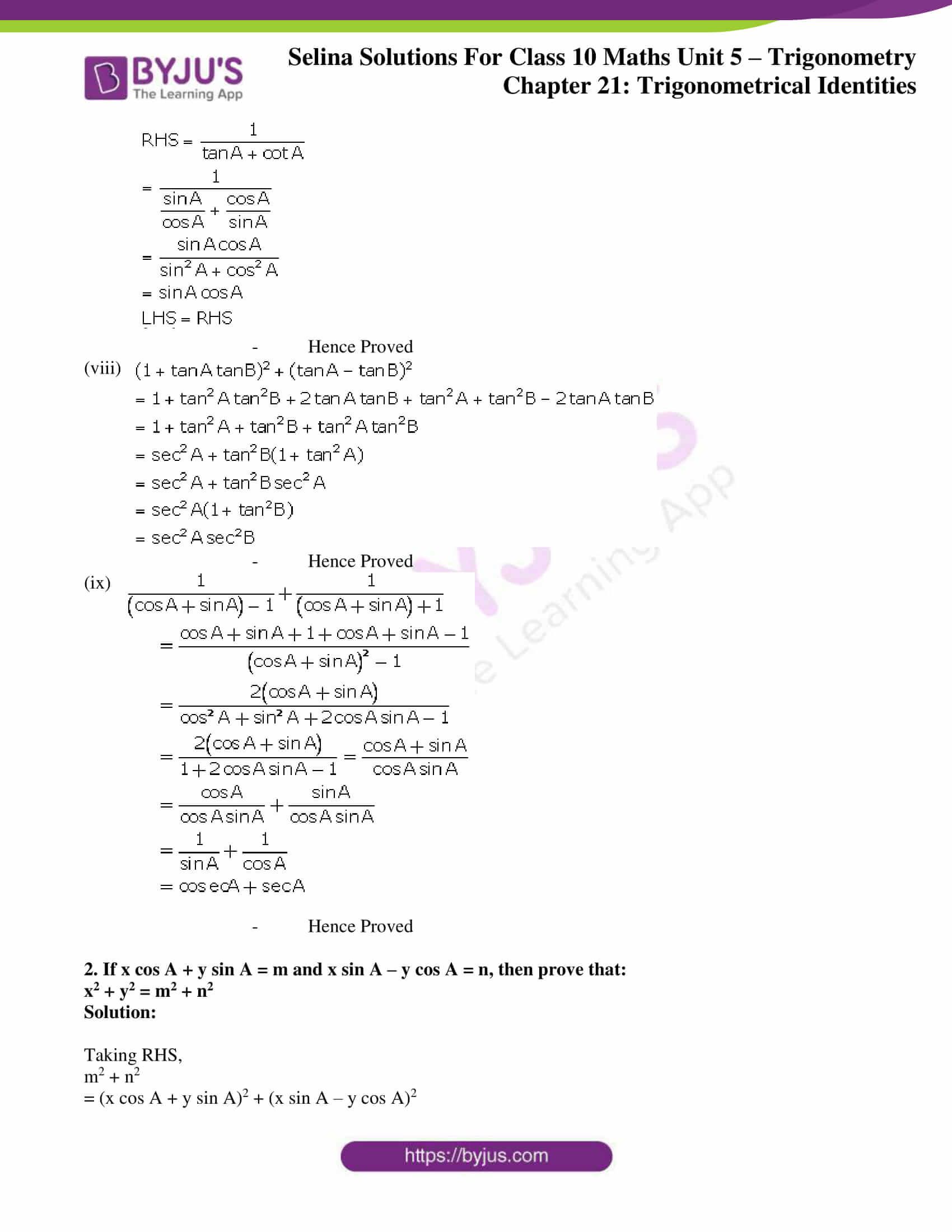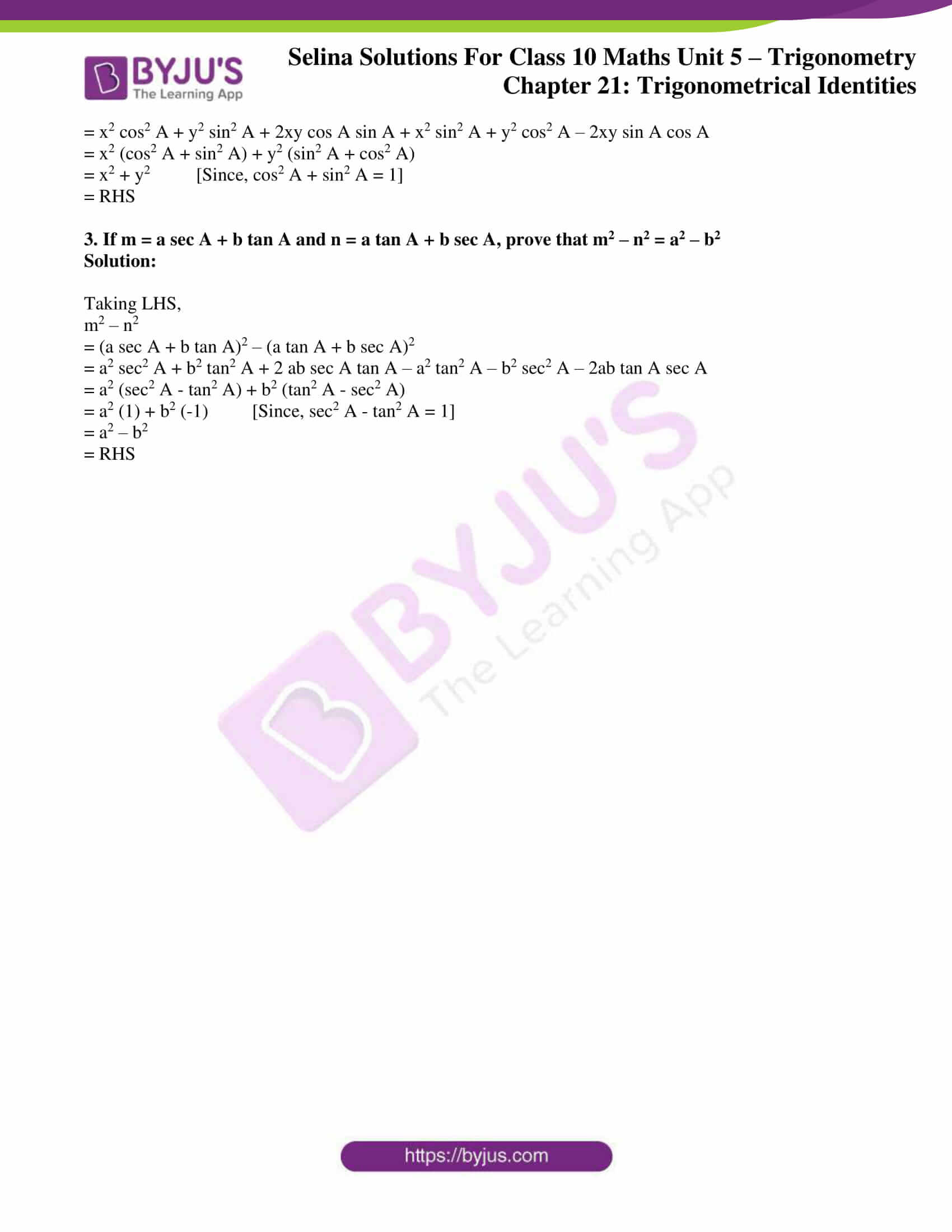### Access Selina Solutions Concise Maths Class 10 Chapter 21 Trigonometrical Identities Exercise 21(B)

1. Prove that: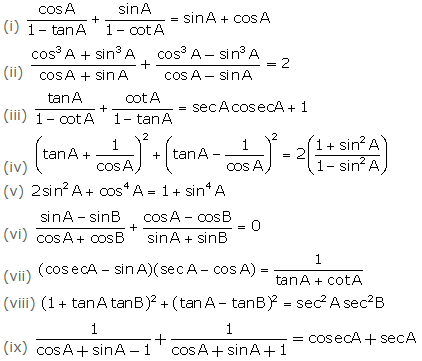Solution: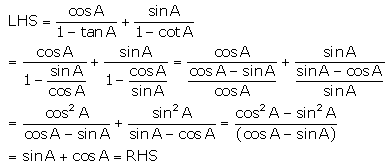(i)

– Hence Proved

(ii) Taking LHS,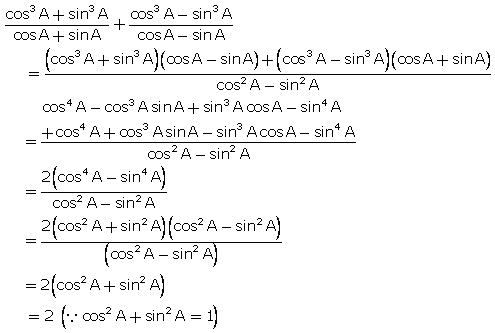– Hence Proved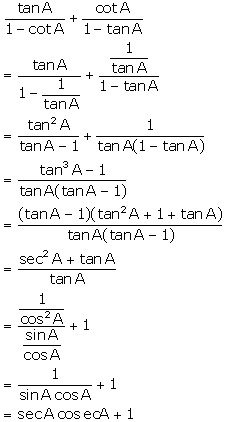(iii)

– Hence Proved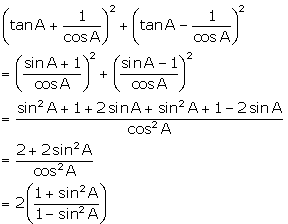(iv)

– Hence Proved

(v) Taking LHS,

2 sin2 A + cos2 A

= 2 sin2 A + (1 – sin2 A)2

= 2 sin2 A+ 1 + sin4 A – 2 sin2 A

= 1 + sin4 A = RHS

– Hence Proved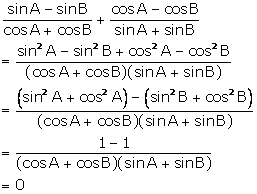(vi)

– Hence Proved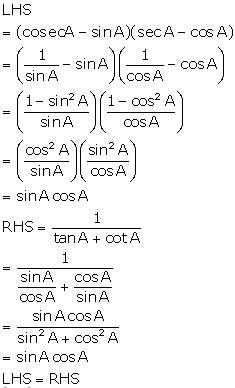(vii)– Hence Proved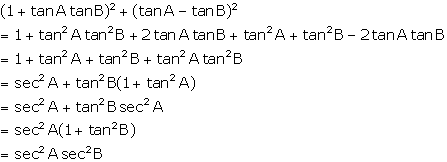(viii)

– Hence Proved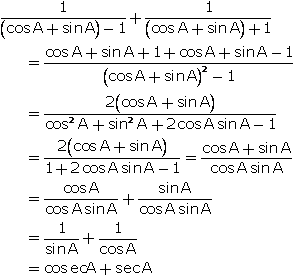(ix)

– Hence Proved

2. If x cos A + y sin A = m and x sin A – y cos A = n, then prove that:

x2 + y2 = m2 + n2

Solution:

Taking RHS,

m2 + n2

= (x cos A + y sin A)2 + (x sin A – y cos A)2

= x2 cos2 A + y2 sin2 A + 2xy cos A sin A + x2 sin2 A + y2 cos2 A – 2xy sin A cos A

= x2 (cos2 A + sin2 A) + y2 (sin2 A + cos2 A)

= x2 + y2 [Since, cos2 A + sin2 A = 1]

= RHS

3. If m = a sec A + b tan A and n = a tan A + b sec A, prove that m2 – n2 = a2 – b2

Solution:

Taking LHS,

m2 – n2

= (a sec A + b tan A)2 – (a tan A + b sec A)2

= a2 sec2 A + b2 tan2 A + 2 ab sec A tan A – a2 tan2 A – b2 sec2 A – 2ab tan A sec A

= a2 (sec2 A – tan2 A) + b2 (tan2 A – sec2 A)

= a2 (1) + b2 (-1) [Since, sec2 A – tan2 A = 1]

= a2 – b2

= RHS